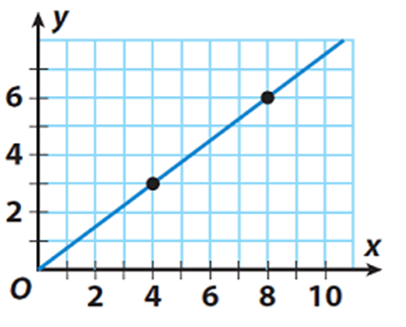SherpaMaths

>

GCSE

Rates of Change

Question

# How do you find the rate of change?

1 year ago

·

126 Replies

·

4413 views

V

Vickie Shanahan

D
Darcy Peake

The rate of change refers to how much something for example distance is changing in a specific unit of time. In order to work out the rate of change, you must divide the thing that is changing with how much it has changed in such a unit of time.

U
Unai Llona Carbajo

Derivatives are the best tool, but not for everyone... Considering two variables (distance and time, for example), you can also take the first and last value of both in a certain process, subtract the ones from the same variable (e.g. last and first distances of the bus from your position) and divide the subtractions of different variables. By doing this with position/distance and time, the rate of change you get is the speed of the object you're analyzing!

Fyruz N Verified Sherpa Tutor ✓

My teaching is based on the individual learning system of the student.

rate of change can be found by using the equation - Difference in Y/ Difference in X

I'm available for 1:1 private online tuition!

N
NEHA GUPTA

By the help of differentiation

H
Hank

If we get the explicit equation, we could just differentiate that wrt the variable. If we don’t, we can estimate it by calculating the change on the y-axis and dividing it by the change on the x-axis.

C
Clara Marchese

The rate of change is given by the division of the output of a system y by the change in the x values, which is the input

G
George Asquith

The rate of change is how much one quantity changes per unit of another amount. Normally this is how much y changes for every set amount that x increases or decreases. This is the same as the gradient (in straight lines gradient is fixed and is shown as m in y=mx+c, in other graphs, the gradient changes). This can be calculated by having two points each with x and y coordinates. y1-y2 divded by x1-x2.

M

How do find the rate of change

S
Stavros Savva

Divide the change of one variable with the change of the other variable

Y
Yunho Kwon

Linear functions have a constant rate of change which is represented as the gradient of the graph.

It is simply the change of y-values with respect to x-values i.e. (y1-y2) / (x1-x2) where (x1,y1), (x2,y2) are the coordinates

L
Leon Cragg

the change in y-values by the change in x-values.

C
Charlie

To find the rate of change, you must divide the change in y by the change in x (if using a graph, where y is the vertical axis and x is the horizontal axis).

L
Lewis Lovell

Hello Mrs Vickie Shanahan. There isn't much context to your question here, so I'll keep my response as general as possible:

The rate of change describes a relationship between a dependent and an independent variable. For example, the rate of change of velocity with time or the rate of change of temperature with distance.

In a simple mathematical case, we'd write this as the change in the dependent variable (temperature, dT) over a fixed interval of the independent variable (distance, dx)

Which is mathematically written as dT/dx, or as shown in the graph, dy/dx.It is then clear that for the linear relationship in the above graph, the rate of change is dy/dx = (y1-y2)/(x2-x1) = (6-3)/(8-4).

A
Amber Cummins

There are a couple of ways to find this. If your data is displayed in graph format, you can find the rate of change by dividing the change in y-values by the change in x-values. If you are talking about the instantaneous rate of change (this is called the derivative of a function) then we need to look further at the function itself.

Fiaraz I Verified Sherpa Tutor ✓

Qualified Teacher, Tutor, Examiner, STEM ambassador and Xprize Alumni

3 reviews

if you are given a graph then draw a tangent as accurately as you can(doesn't matter if its perfect) and use change in y divided by change in x for your straight line to get the gradient which is the rate of change. just watch your units it will ber per second or per minute, something like that depending on the experiment

I'm available for 1:1 private online tuition!